Choose Language Hide Translation Barltw
Staff (Retired)
Reliability regression with binary response data (probit analysis) with JMP

Many readers may be familiar with the broad spectrum of reliability platforms and analysis methods for reliability-centric problems available in JMP. The methods an engineer will select – whether to solve a problem, improve a system or gain a deeper understanding of a failure mechanism – are dependent on many things. These dependencies could include whether the system or unit under study is repairable or non-repairable. Is the data censored, and if so, is it right-, interval-, or left- censored? What if there are no failures? How can historical data on the same or similar component be used to augment understanding?

I’d like to address a data issue specific to the response variable. The Reliability Regression with Binary Response technique can be a useful addition to the tools that reliability engineers or medical researchers use to answer critical business and health-related questions. For instance, when the response variable is simply counts of failures, rather than the much more commonly occurring response that is continuous in nature, alternate analytical procedures should be used. For example, say you are testing cell phone for damage due to dropping phone onto floor. You may test 25 phones each at various heights above the floor, e.g. 5 feet, 8 feet etc. Then you simply record the number of failures (damaged phones) per sample set. In a health related field, you may want to test the efficacy of a new drug at differing dosages, or compare different treatment types and record the patient survival counts.

The purpose of this blog post is to help you understand how you can perform regression analysis on reliability and survival data that has counts as the response. This is known as Reliability Regression with Binary Response Data, sometimes referred to as Probit Analysis. The data in Table 1 is a simple example from a class I attended at the University of Michigan a number of years ago. The study is focused on evaluating a new formulation of concrete to determine failure probabilities based on various load levels (stress factor). A failure is defined as a crack of some specified minimum length. Some questions we would like to answer include the following:

• For a given load, say 4,500 lbs., what percent will fail?
• What load will cause 10%, 25%, and 50% of the concrete sections to crack?
• What is the 95% confidence interval that traps the true load where 50% of the concrete sections fail?
•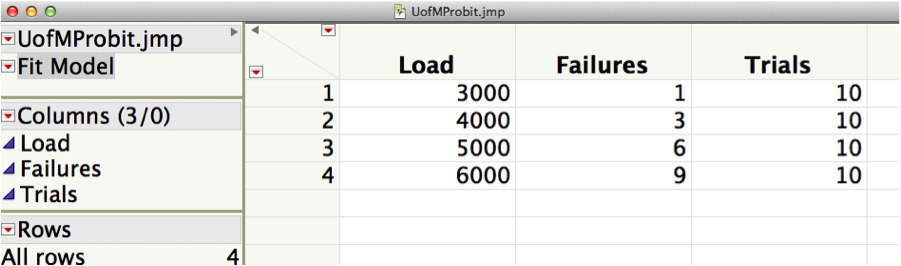The data contains three columns. The Load column is the amount of pressure, in pounds, applied to the concrete sections. Trials are the number of sections tested, and Failures is the number of sections that failed as a result of crack development under the applied pressure. We will use JMP’s Fit Model Platform to perform the analysis. Depending on the distribution selection you choose to analyze your data with, I refer you to Table 2 below which will assist you in selecting the correct Link Function and appropriate transformation, if required, for your x variable.

Distribution
Transformation on X
Sev
Comploglog
None
Weibull
Comploglog
Log
Normal
Probit
None
Lognormal
Probit
Log
Logistic
Logit
None
Loglogistic
Logit
Log

Table 2: Depending on your distribution, this table will guide you to the appropriate Link and Transformation selections in the Fit Model Dialog.

Open the data table and click the JMP Analyze menu, then select Fit Model. Once the dialog window opens, select the Load and Trials column and add to the Y dialog. Add Load as a model effect, and then highlight load in the Construct Model Effects dialog, click the red triangle next to Transform and select Log. Your model effect should now read Log(Load) as seen in the completed Fit Model dialog screen below. Select Generalized Linear Model for Personality, Binomial for Distribution since we are dealing with counts and Comp LogLog for the Link Function since we are using a Weibull fit for this example.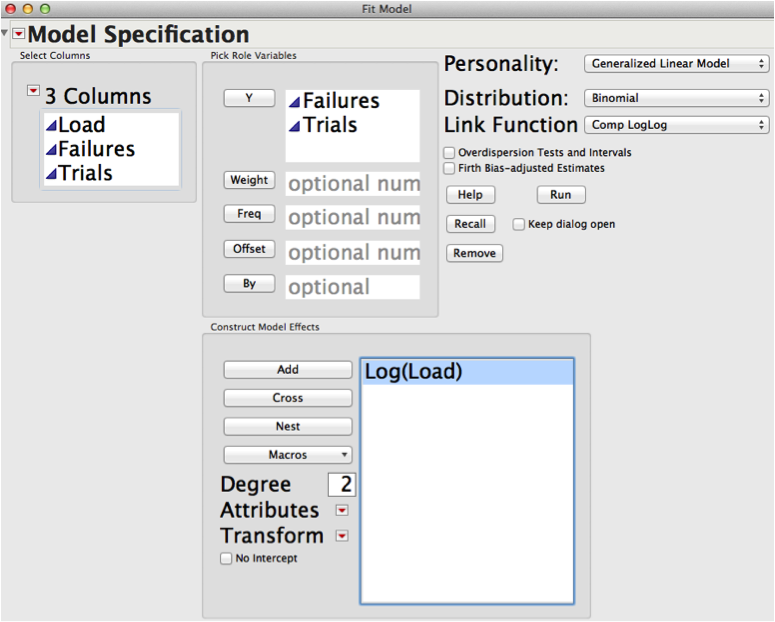Figure 1: Completed Fit Model Dialog for fitting a Weibull in our example.

Next select Run. You will see the output in Figure 2: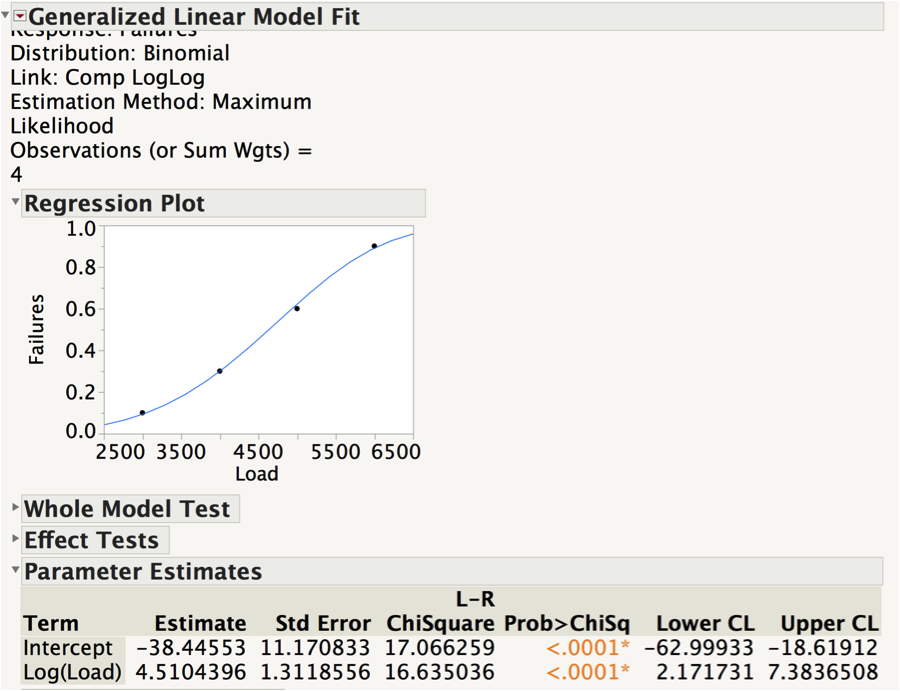Figure 2: Initial output with Regression Plot and associated output. Note the Log(Load) parameter estimate of 4.51 is the Weibull shape parameter.

So now let’s begin to answer the questions we posed at the beginning. To find out what percent of sections fail at a load of 4,500 lbs, go to the red triangle at the top next to the output heading Generalized Linear Model Fit. Select Profilers > Profiler. See Figure 3. Scroll down in the report window and drag the vertical red dashed line to select 4,500 for load, or highlight the load value on the x-axis and type in 4,500. You will see that at a load of 4,500 pounds, we can expect a 45% failure rate. The associated confidence interval may be of interest as well. With this current sample, results could range from as small as 29% up to as high as 65%.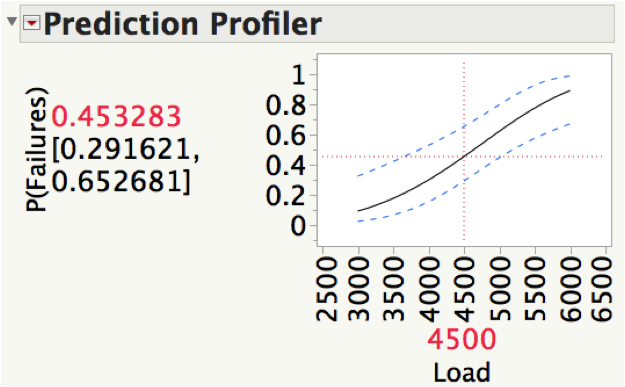Figure 3: Prediction Profiler with a load of 4,500 pounds.

Now, to find out what load will cause 10%, 25%, and 50% of the concrete sections to crack, we again go to the red triangle at the top of the report and select Inverse Prediction. You will see the following dialog in Figure 4. Type in 0.1, 0.25 and .50 to obtain results for 10, 25 and 50 percent, respectively.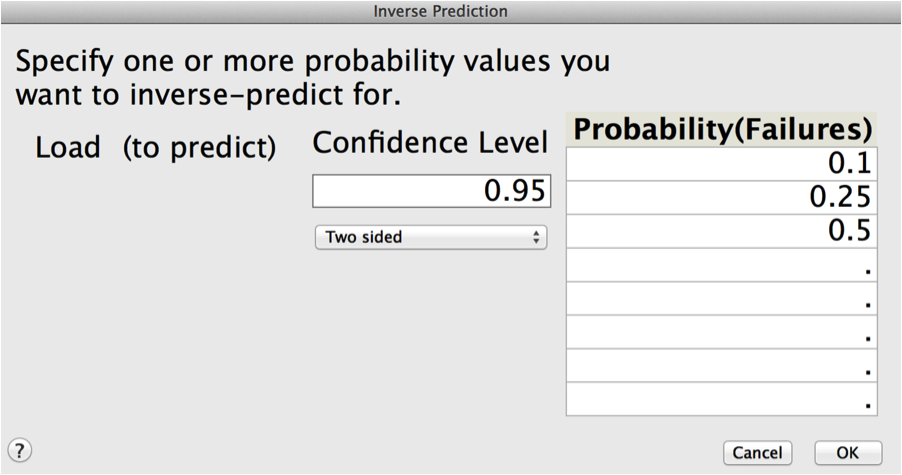Figure 4: Dialog for Inverse Prediction

Scroll down in the report where you will find the Inverse Prediction output. See Figure 5. The predicted load value, in pounds of pressure, for the B10 is 3055, B25 is 3817and B50 is 4639. A corresponding plot, which includes a visual representation of the confidence intervals, is also provided.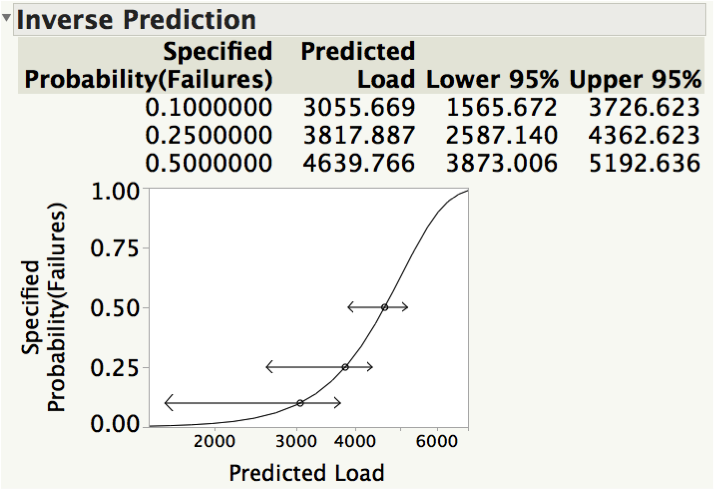Figure 5: Inverse Prediction output.

Finally, we would like to find the 95% confidence interval that traps the true load where 50% of the concrete sections fail. Again, refer to the Inverse Prediction output in figure 5. We find that a lower bound of 3,873 up to an upper bound of 5,192 traps 95% of the true load where 50% of the sections fail.

JMP has numerous capabilities for reliability analysis, with many dedicated platforms such as Life Distribution, Reliability Growth and Reliability Block Diagram, to name just a few. However, as you can see here, you can perform other reliability and survival analysis methods that using other JMP analysis platforms.

Article Labels

There are no labels assigned to this post.

Article Tags